# Statistics

Explore Statisitcs, which deals with the collection, classification, analysis, presentation, and interpretation of data.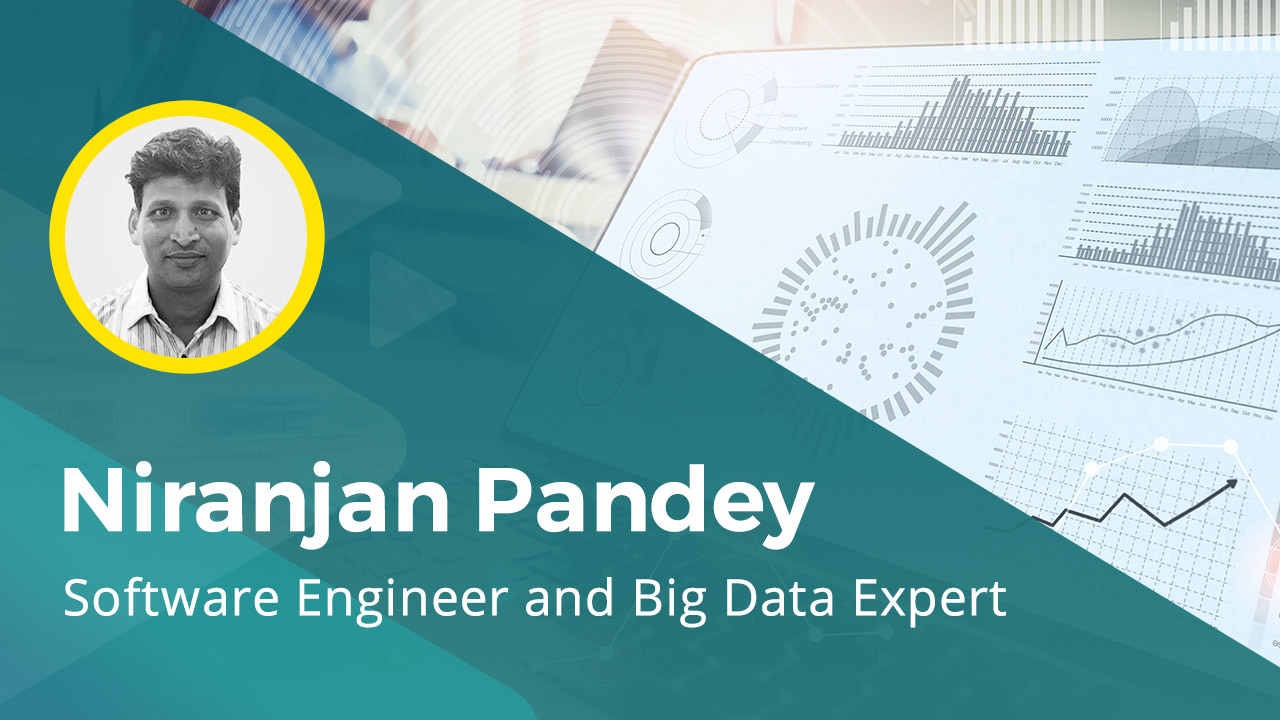Explore the features of simple and multiple regression, implement simple and multiple regression models, explore the concept of gradient descent and regularization and the different types of gradient descent and regularization.We will also learn to implement different types of gradient descent and regularization using prominent Python libraries.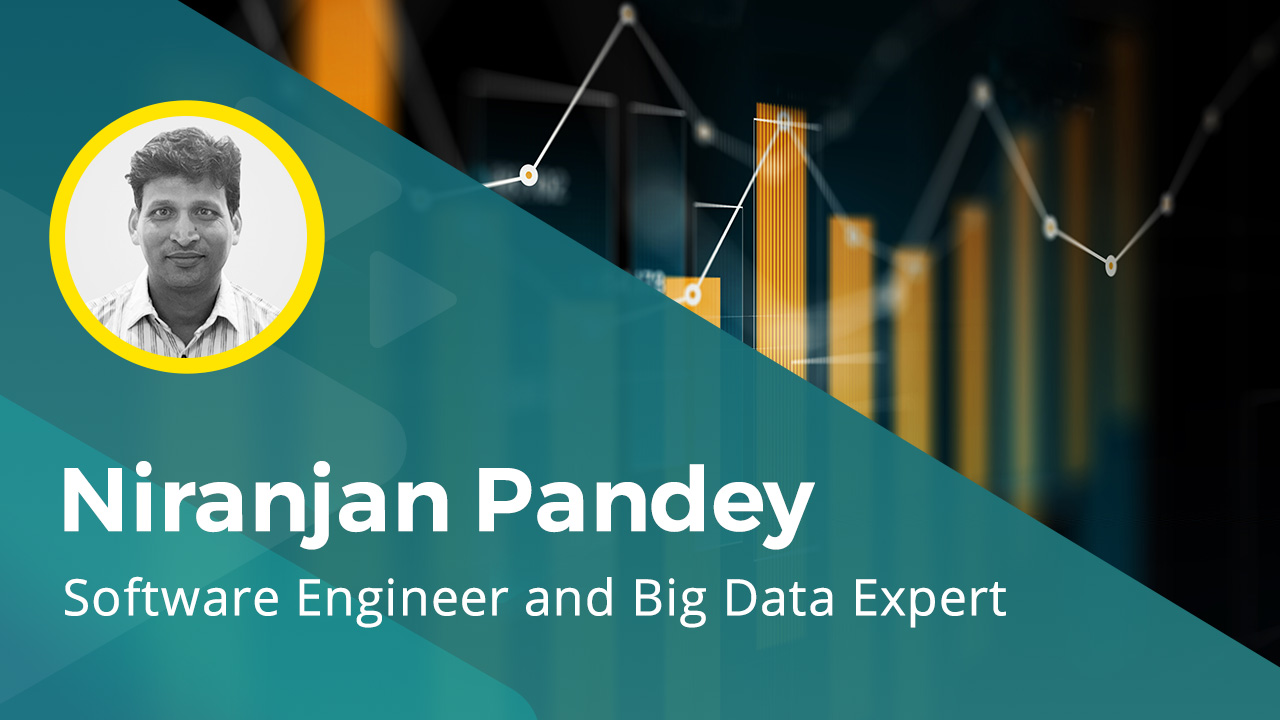Explore the concept of linear models, the classification of linear models, and the prominent statistical approaches that we can use to implement linear models. We will also explore the concept of bias, variance and regularization, how they're used to implement and evaluate linear models and also implement bagging and boosting ensemble algorithms on linear models.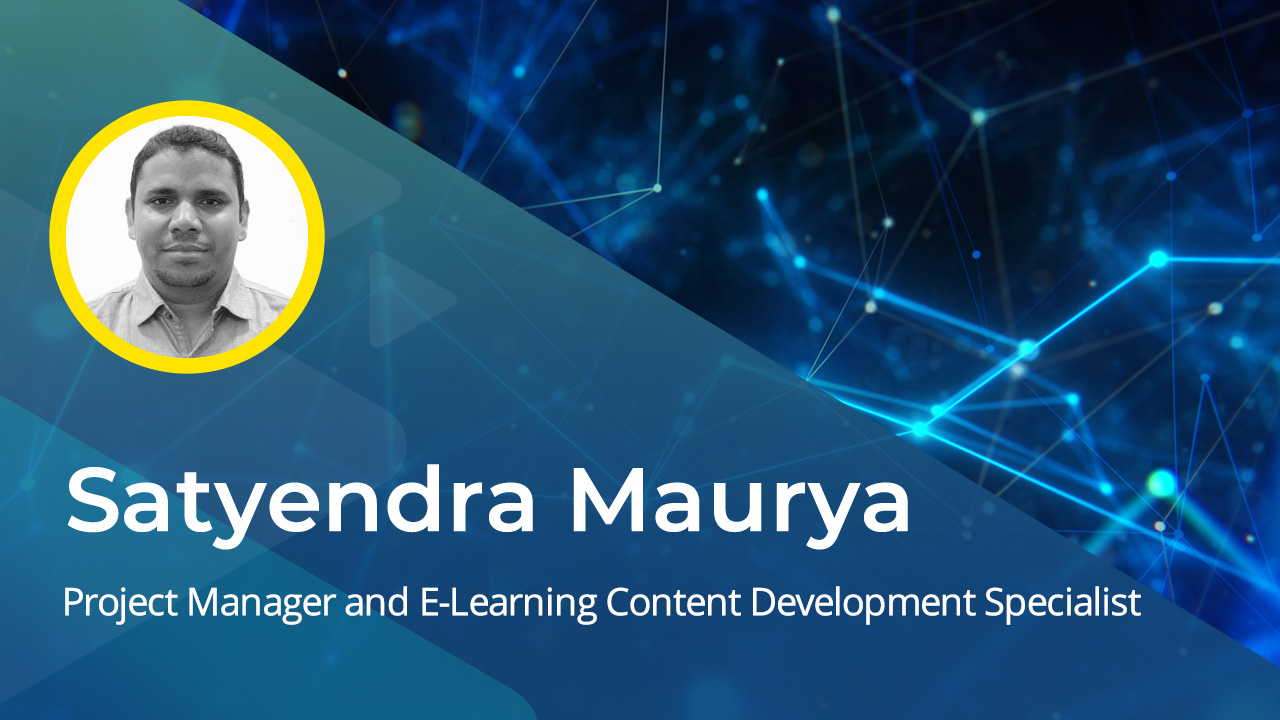Explore the fundamentals of linear algebra, including its characteristics and its role in machine learning. Examine important concepts associated with linear algebra like the class of spaces, types of vector space, vector norms, linear product vector and theorems, and the various operations that can be performed on matrix. How to implement vector arithmetic, vector scalar multiplication, and matrix arithmetic using…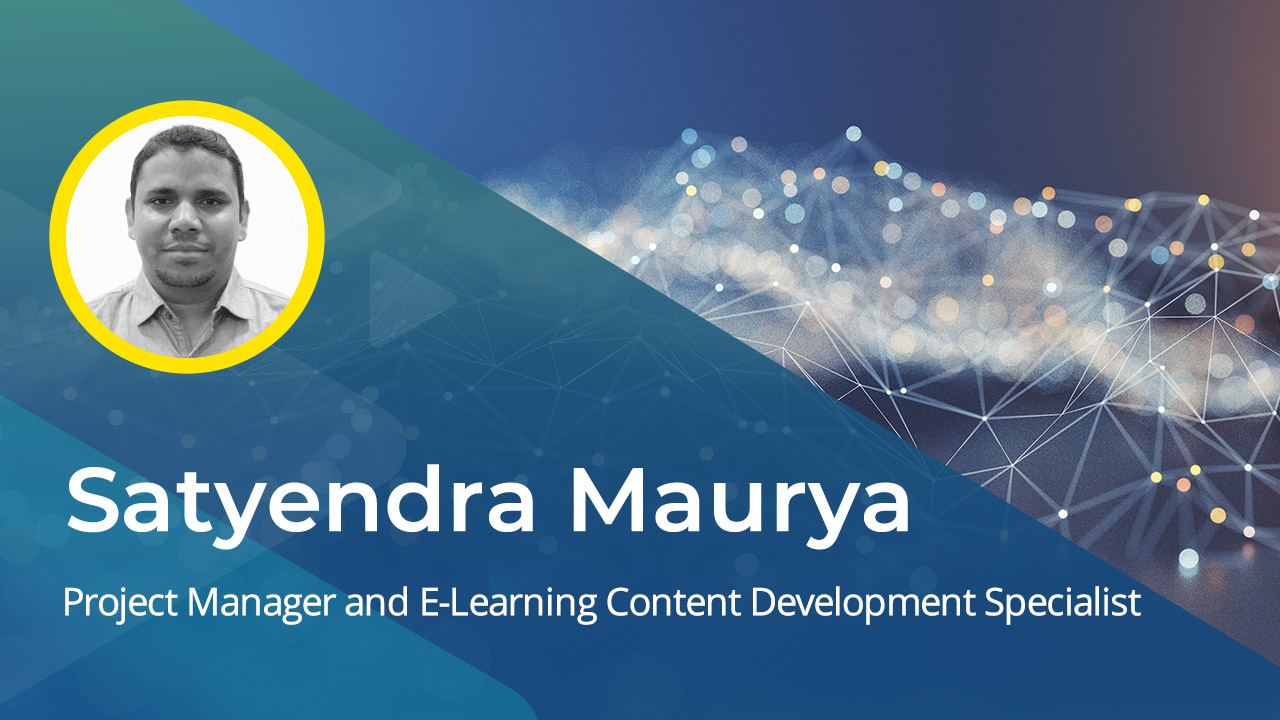Discover how to apply advanced linear algebra and its principles to derive machine learning implementations. Explore PCA, tensors, decomposition, and singular-value decomposition, as well as how to reconstruct a rectangular matrix from singular-value decomposition. The role of statistics and probability, with focus on parameter estimation and Gaussian distribution, is also covered.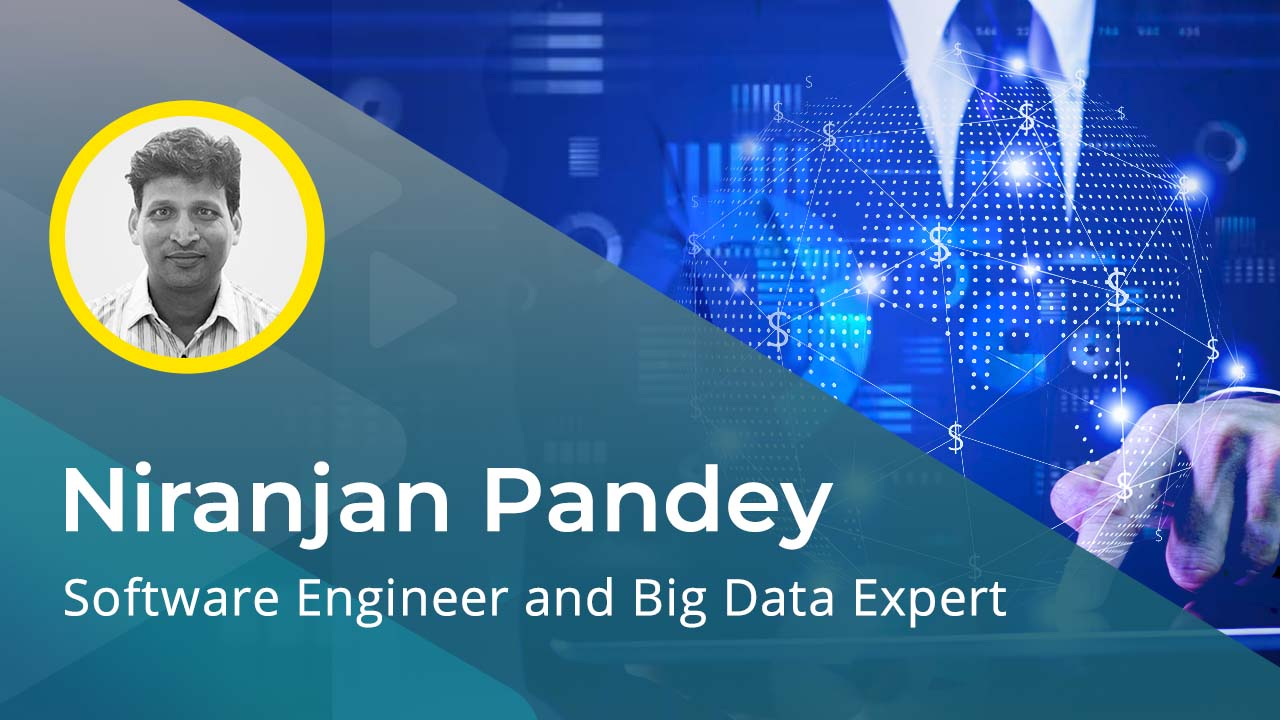Discover the concepts of pushdown automata, Turing machines, and finite transducers. Examine how to identify the limitations and complexities in computation and also how to apply P and NP classes to manage them.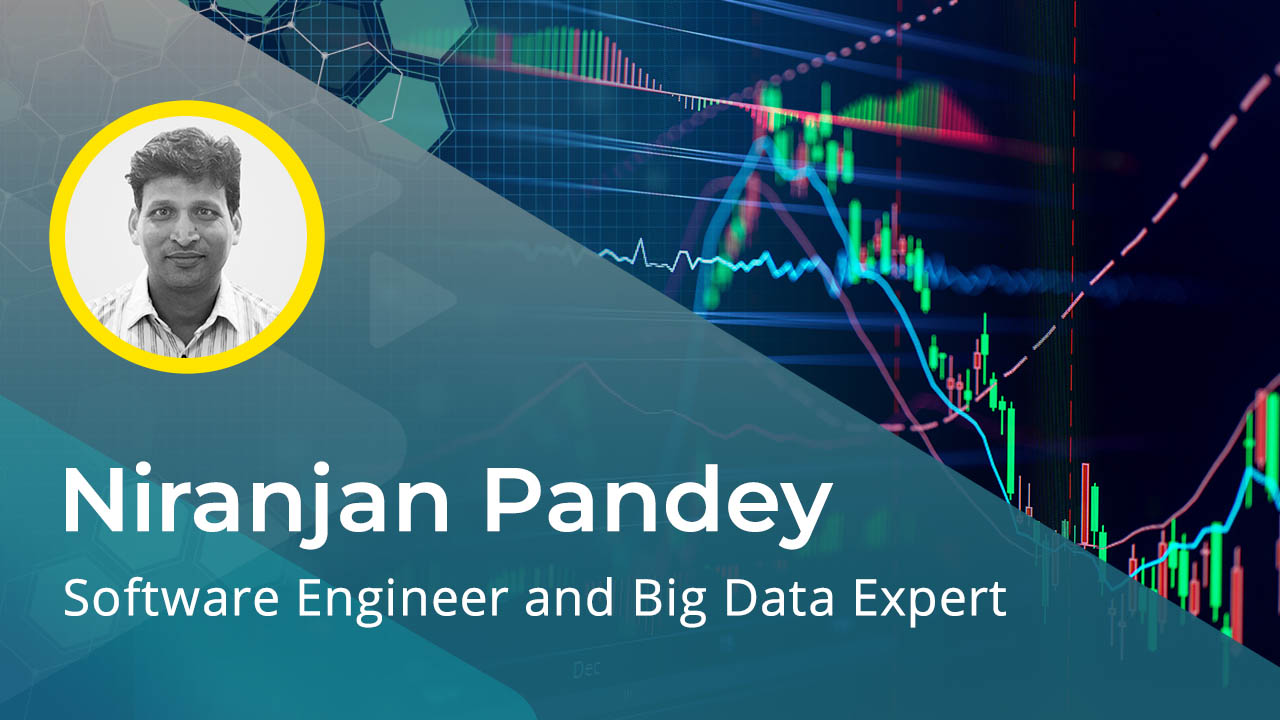Explore the concept of computational theory and its models. Discover how to model and implement computational theory on formal language, automata theory, and context-free grammar.Discover how to use machine learning methods and visualization tools to manage anomalies and improvise data for better data insights and accuracy.Examine statistical and machine learning implementation methods and how to manage anomalies and improvise data for better data insights and accuracy.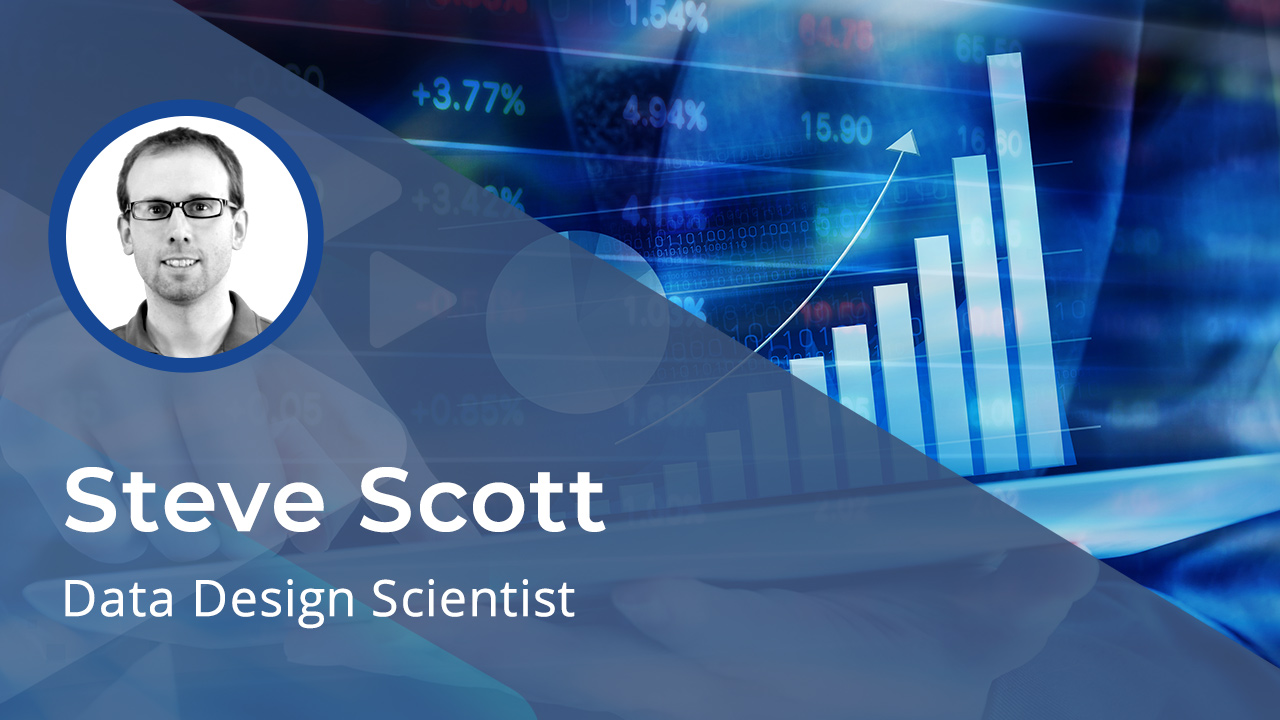Discover how to apply statistical algorithms like PDF, CDF, binomial distribution, and interval estimation for data research. How to implement visualizations to graphically represent the outcomes of data research is also covered.Discover how to produce meaningful information by deriving value from existing data. Explore techniques such as data cleansing, data wrangling, and data categorization.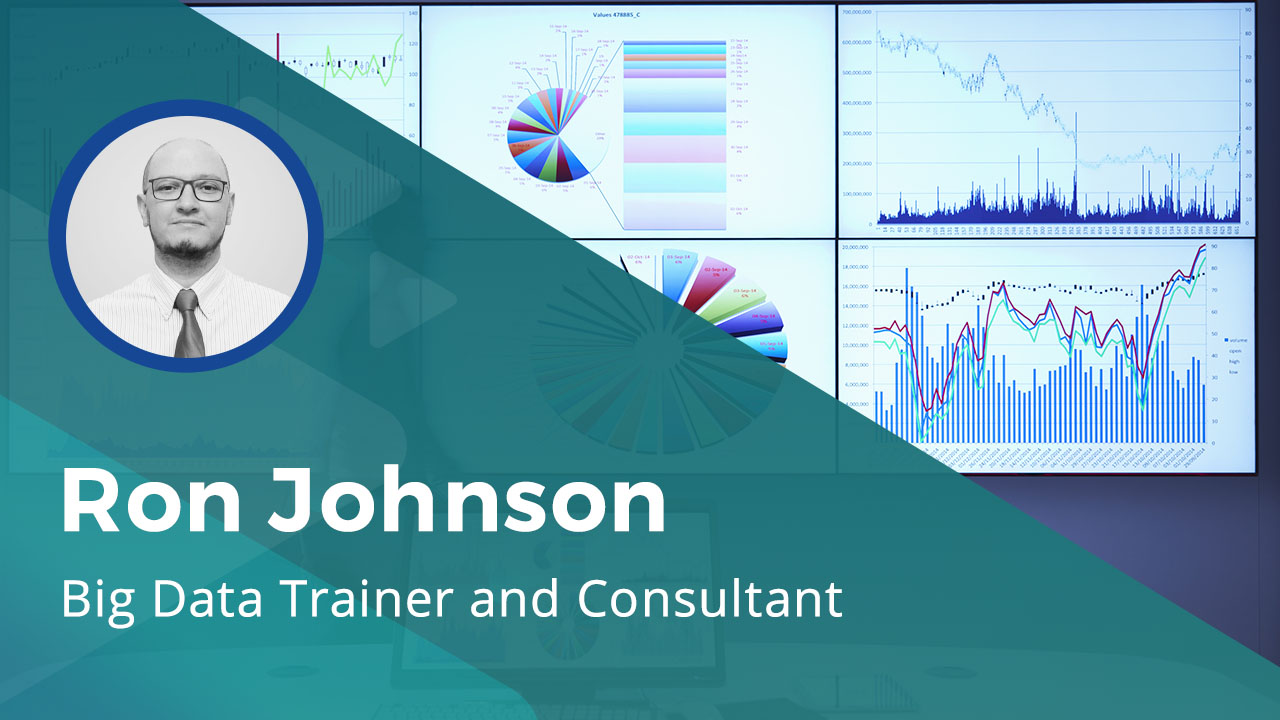Explore data discovery and exploration techniques, such as pivoting, de-identification, analysis, and data tracing.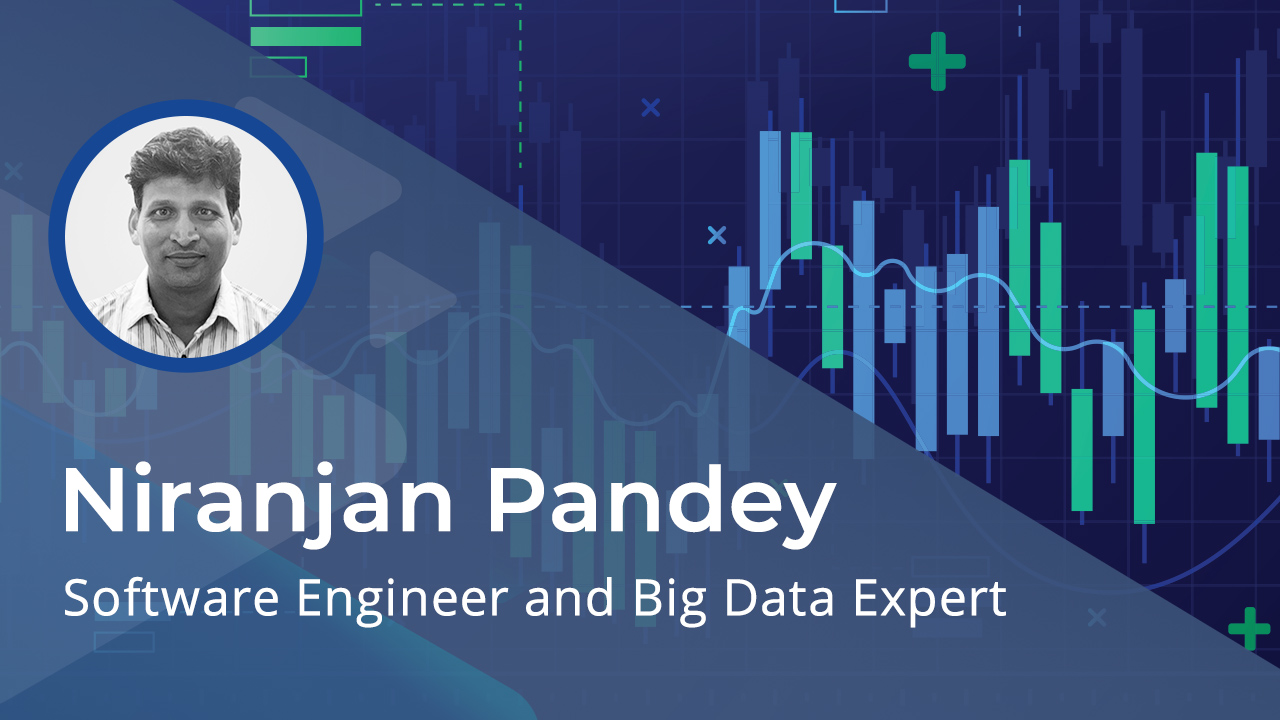To master data science, you must learn the techniques around data research. In this course you will discover how to apply essential data research techniques, including JMP measurement, and how to valuate data using descriptive and inferential methods.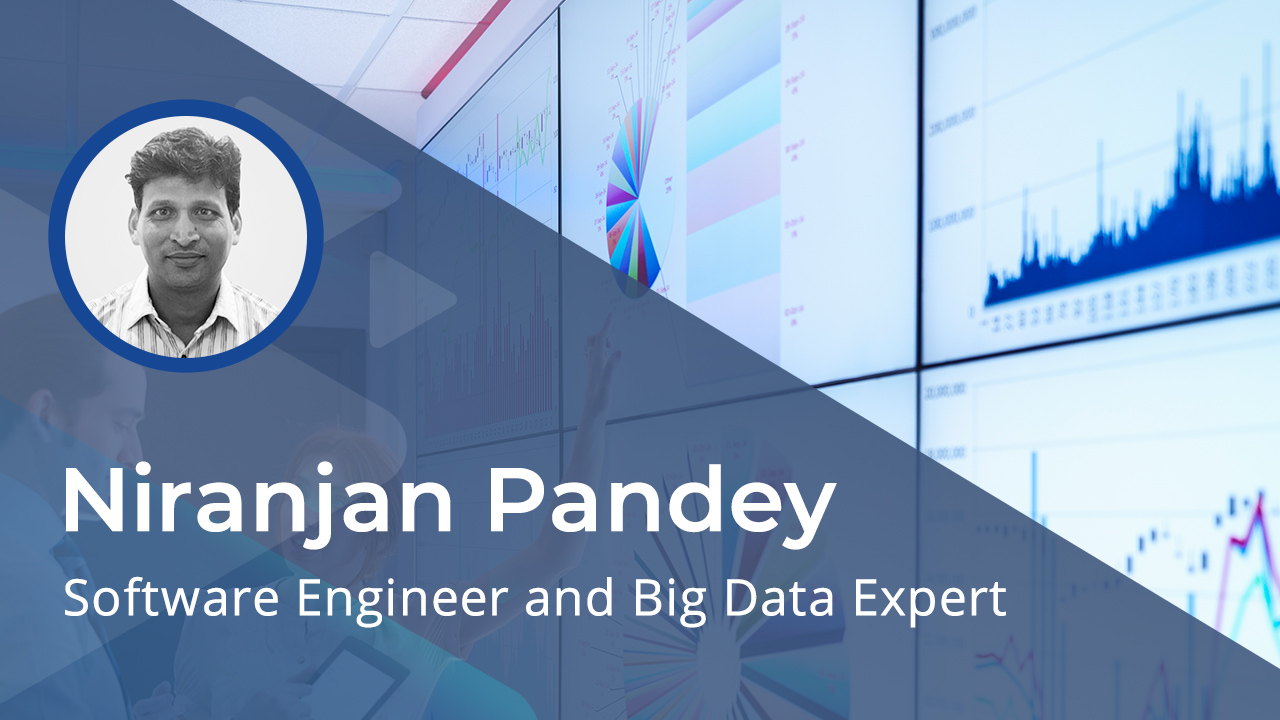To master data science, you must learn the techniques around data research. In this course you will discover how to use data exploration techniques to derive different data dimensions and derive value from the data. How to practically implement data exploration using R, Python, linear algebra, and plots is also covered.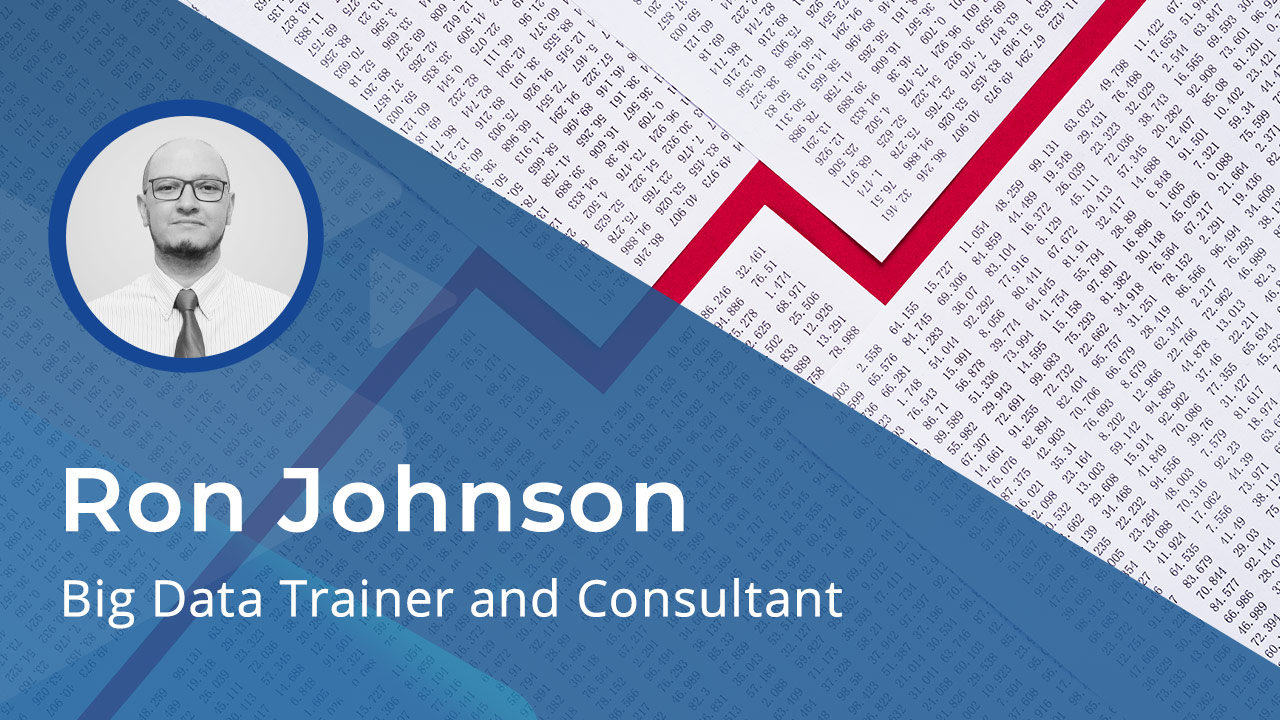To master data science it is important to take raw data and turn that into insights. In this course you will explore the concept of statistical analysis and implement data ingestion using various technologies including NiFi, Sqoop, and Wavefront.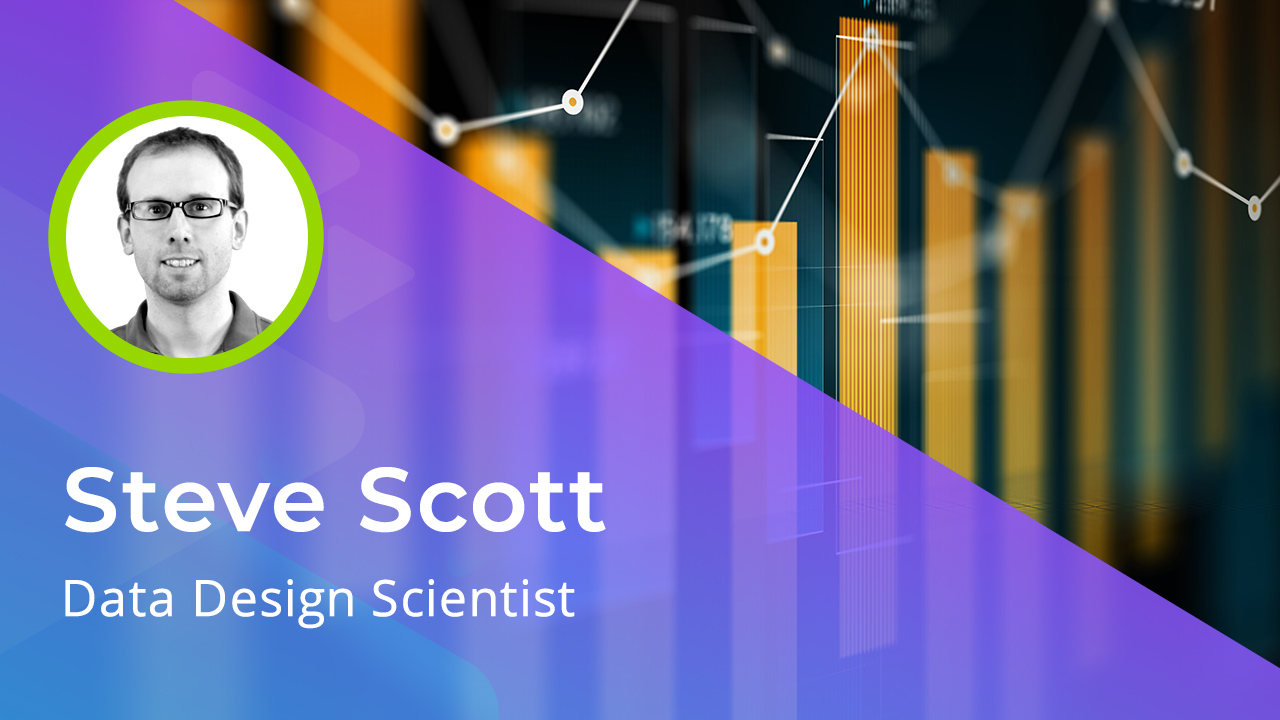Trifacta allows you to discover, wrangle & visualize complex data quickly and has become an essential tool for data science. In this Skillsoft Aspire course, you will discover the essential methods for wrangling data with Trifacta. You will learn how to standardize, format, and filter data and then how to extract and wrangle that data.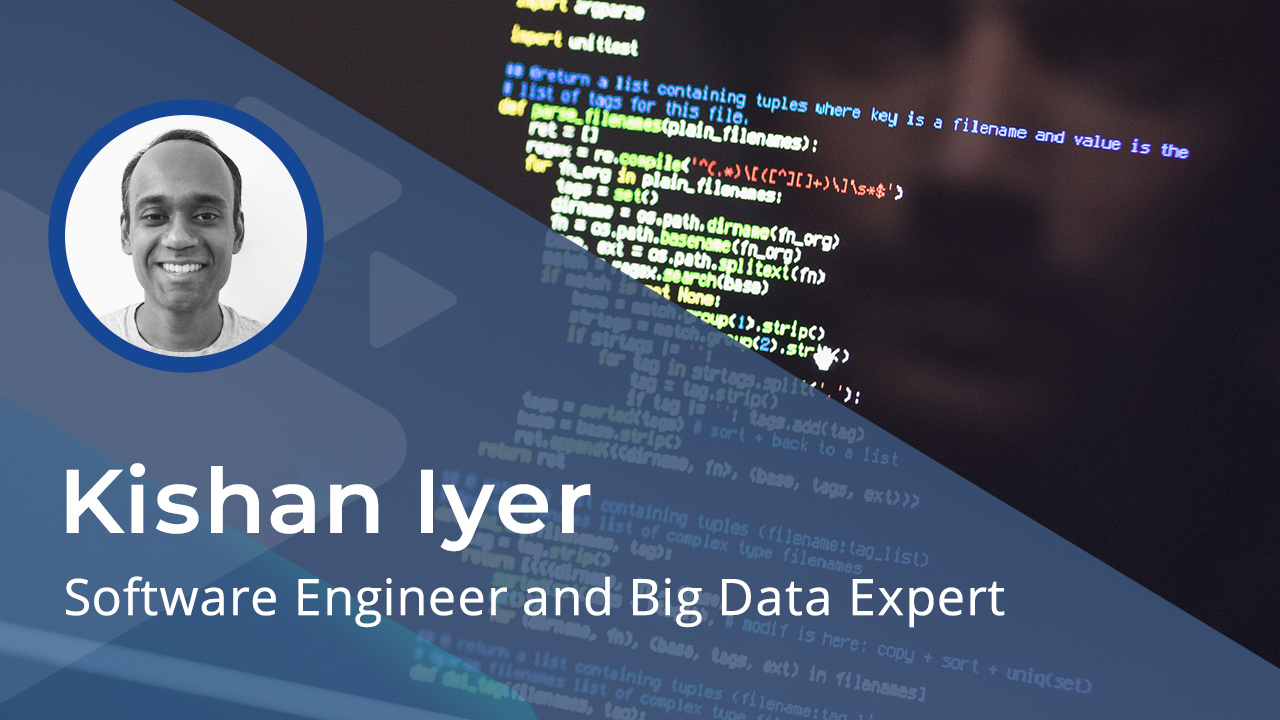On the career path to Data Science, a fundamental understanding of statistics, specifically inferential statistics is required. Explore how different t-tests can be performed using the SciPy library to test hypotheses. How to calculate the skewness and kurtosis of data using SciPy and compute regressions using scikit-learn is also covered.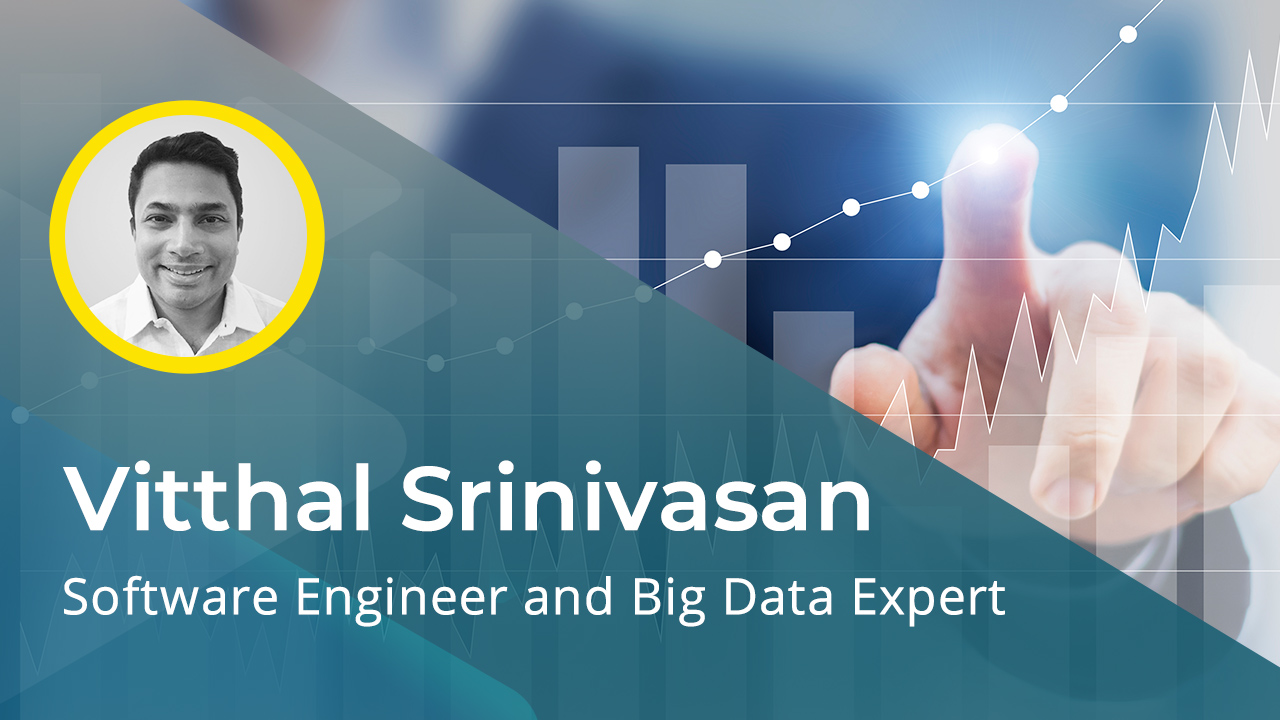On the career path to Data Science, a fundamental understanding of statistics, specifically inferential statistics is required. Inferential statistics go beyond merely describing a dataset and seek to posit and prove or disprove the existence of relationships within the data. In this Skillsoft Aspire course, you will explore hypothesis testing, which finds wide applications in data science.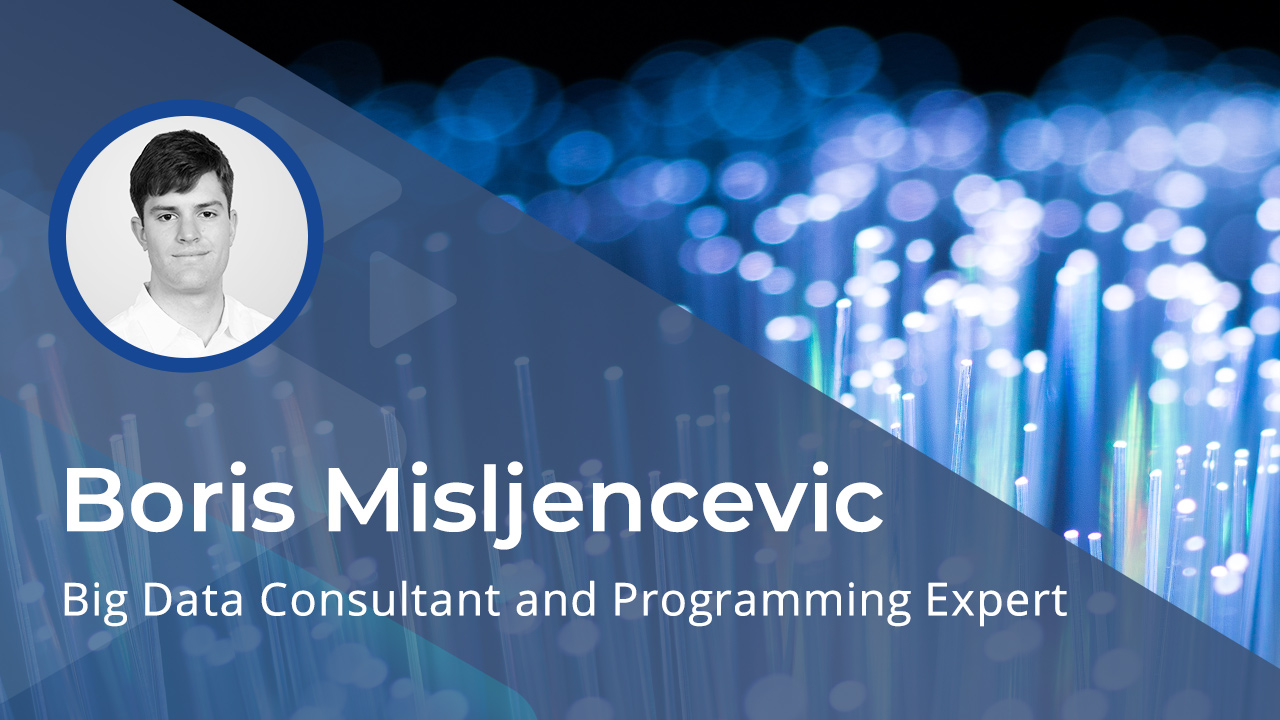As organizations become more data science aware and learn how to collect more data. Taking that data and integrating that into recommendation engines is an essential skill. In this course you will explore how recommendation engines can be created and used to provide recommendations for products and content.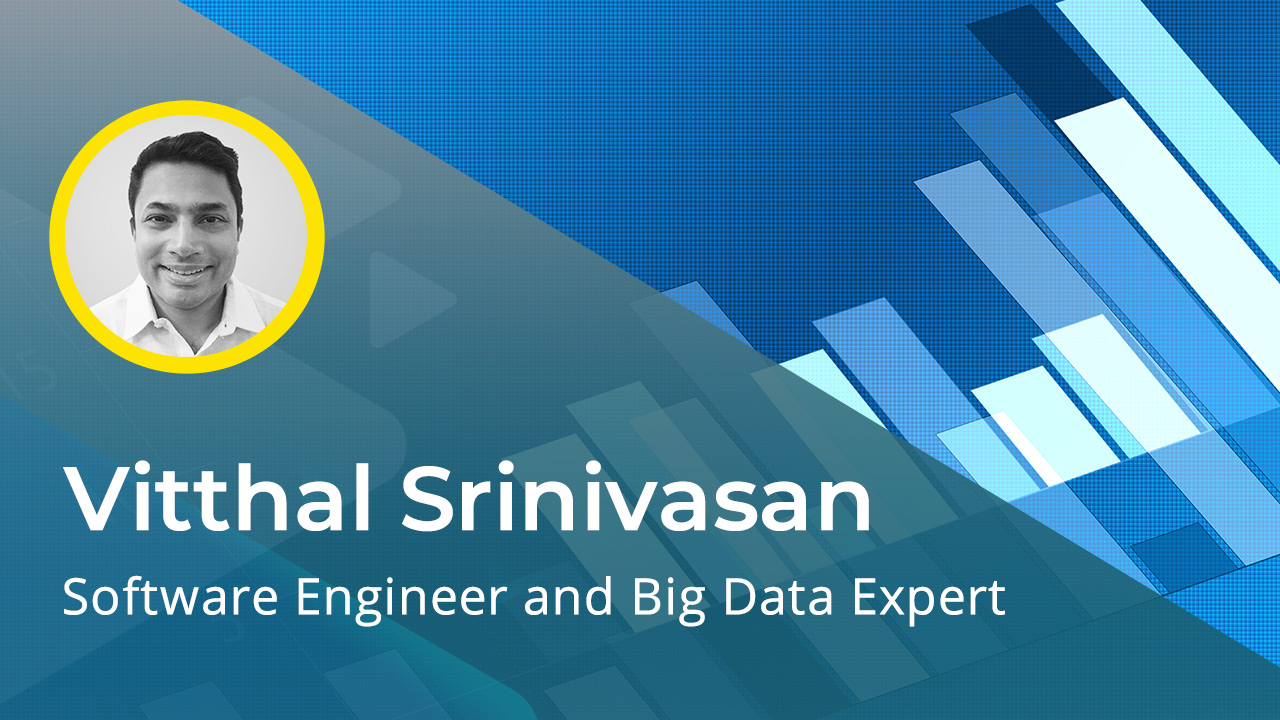On the career path to Data Science, a fundamental understanding of statistics and modeling is required. The goal of all modeling is generalizing as well as possible from a sample to the population of big data as a whole. In thius Skillsoft Aspire course, you will explore the first step in this process, obtaining a representative sample from which meaningful…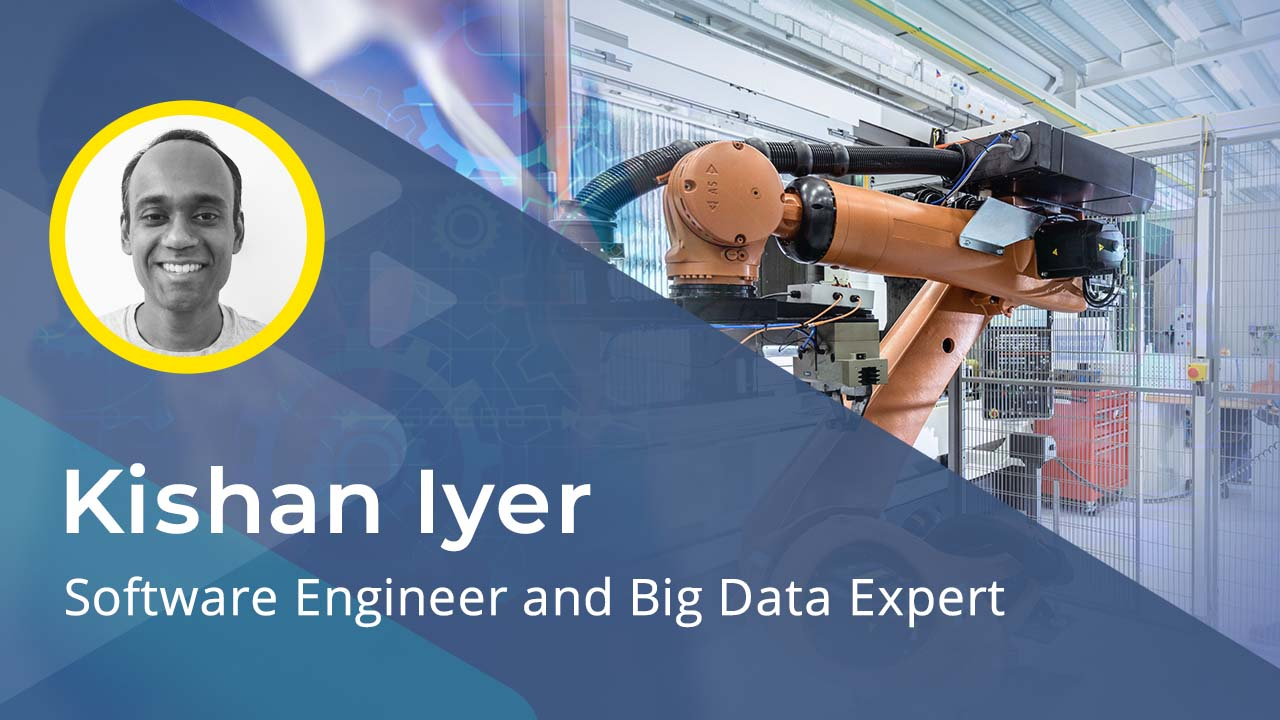There are numerous options available to scale and encode the features and labels in your dataset to get the best out of machine learning algorithms. Explore techniques such as standardizing, normalizing, one-hot encoding, and more.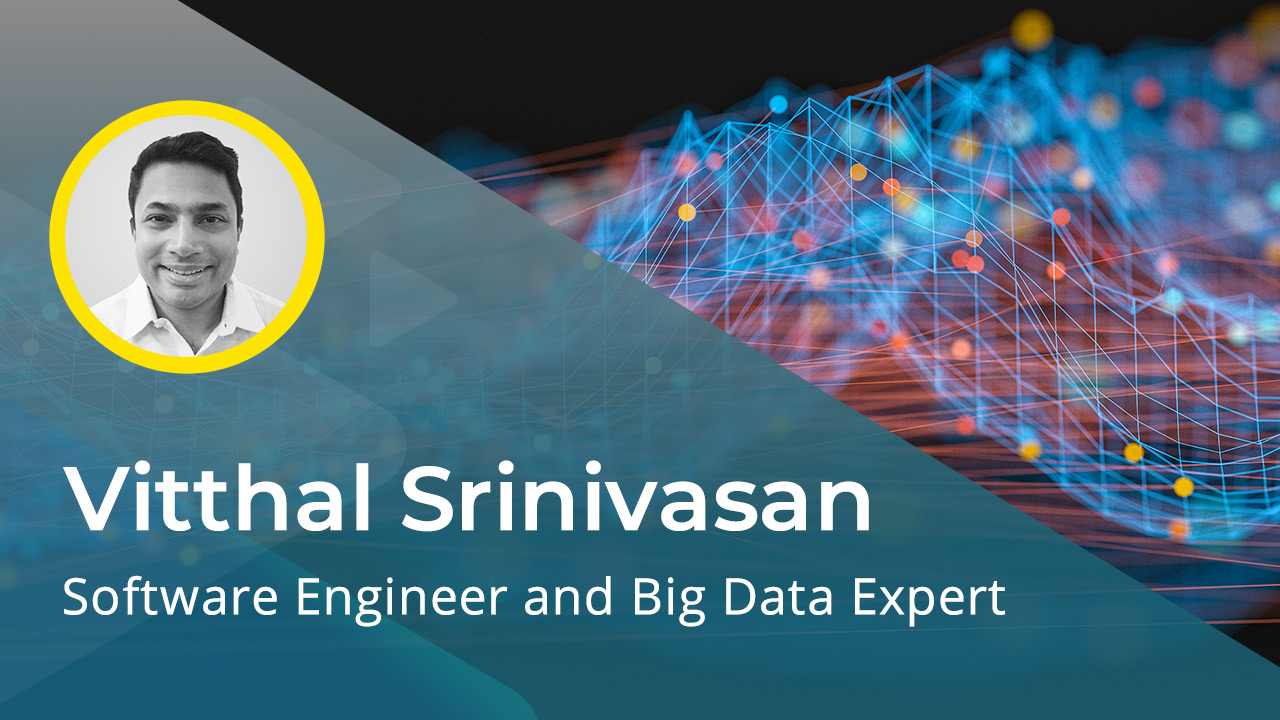On the career path to Data Science, a fundamental understanding and the application of statistics, specifically modeling is required. The goal of all modeling is generalizing as well as possible from a sample to the population as a whole. In this Skillsoft Aspire course, you will explore the first step in this process, obtaining a representative sample from which meaningful…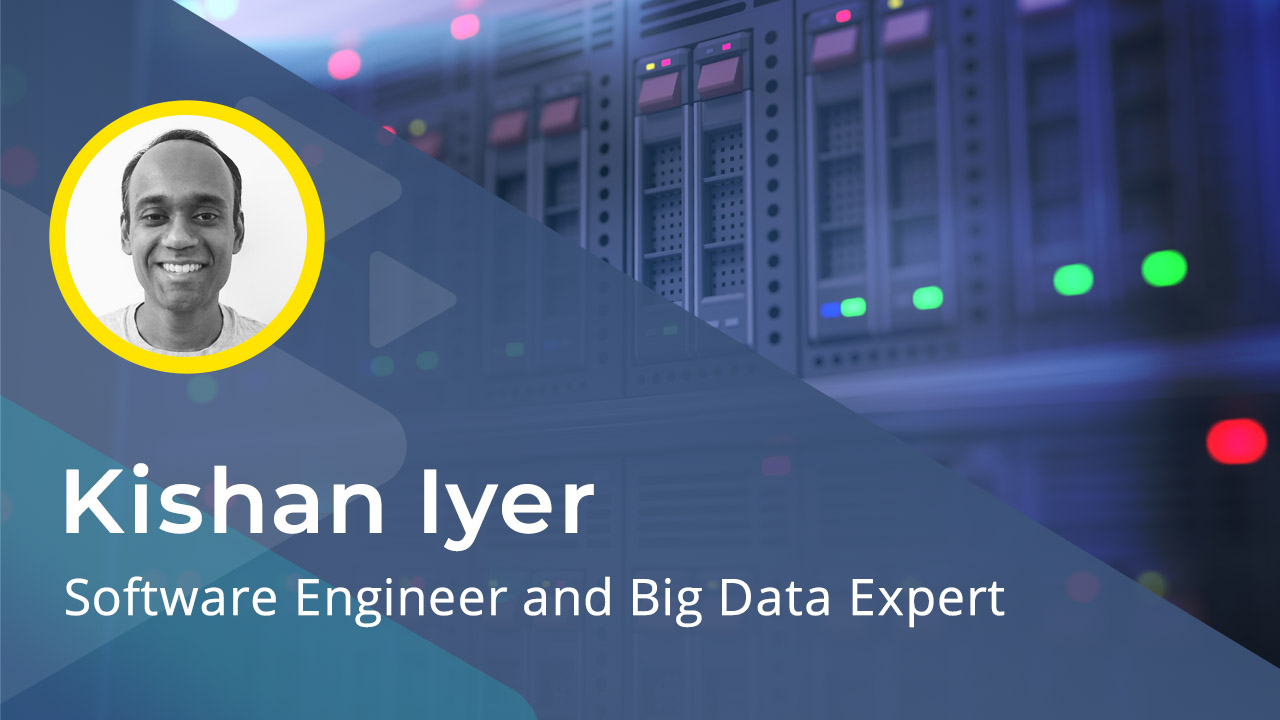Explore how to implement scaling techniques such as standardizing and normalizing on continuous data and label encoding on the target to best use classification models. Examine dimensionality reduction using Principal Component Analysis.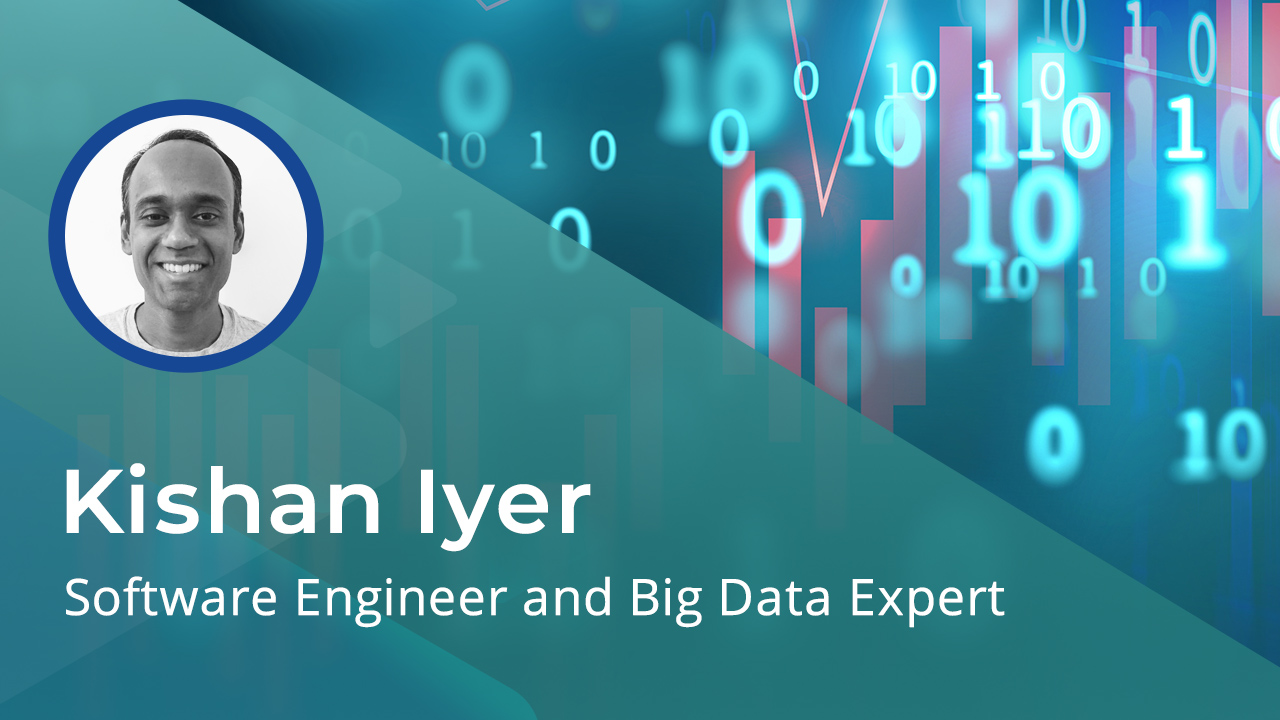On the career path to Data Science, a fundamental understanding and the application and visualization of statistics is required. Discover how to use the NumPy, Pandas, and SciPy libraries to perform various statistical summary operations on real datasets and how to visualize your datasets in the context of these summaries using Matplotlib.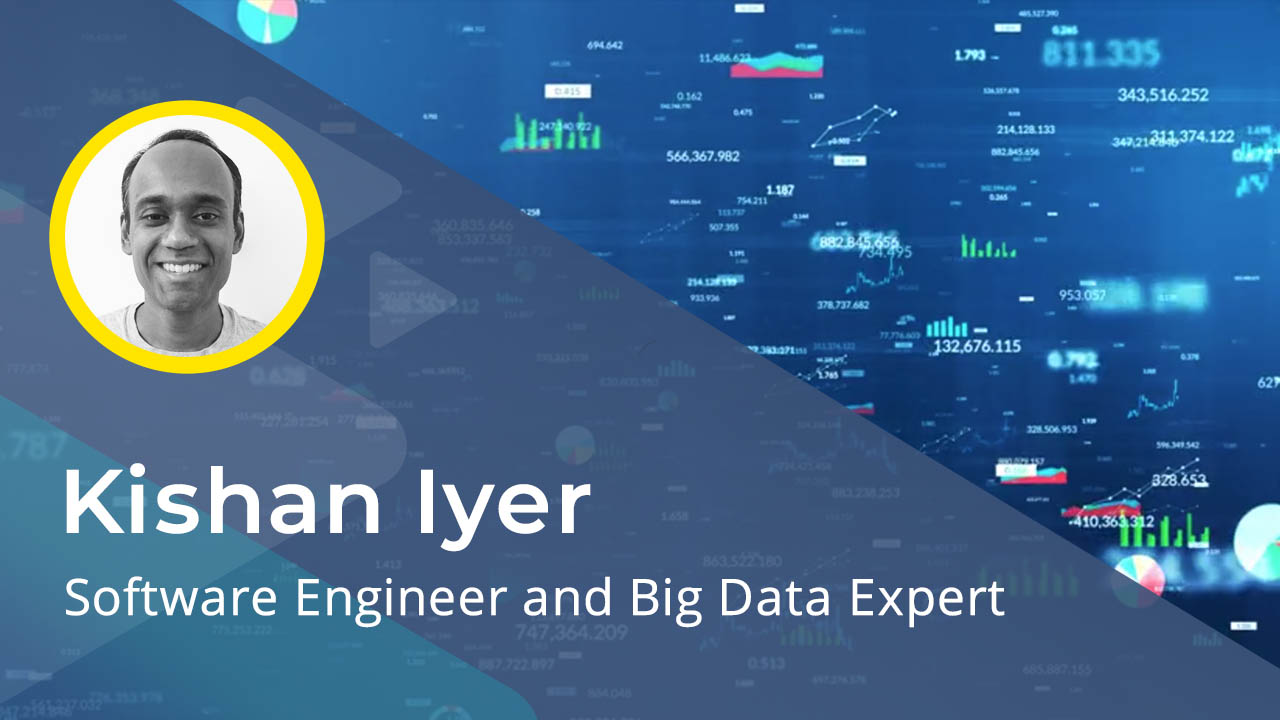Discover how to implement scaling techniques such as standardizing and min-max scaling on continuous data and one-hot encoding on categorical features to improve the performance of linear regression models.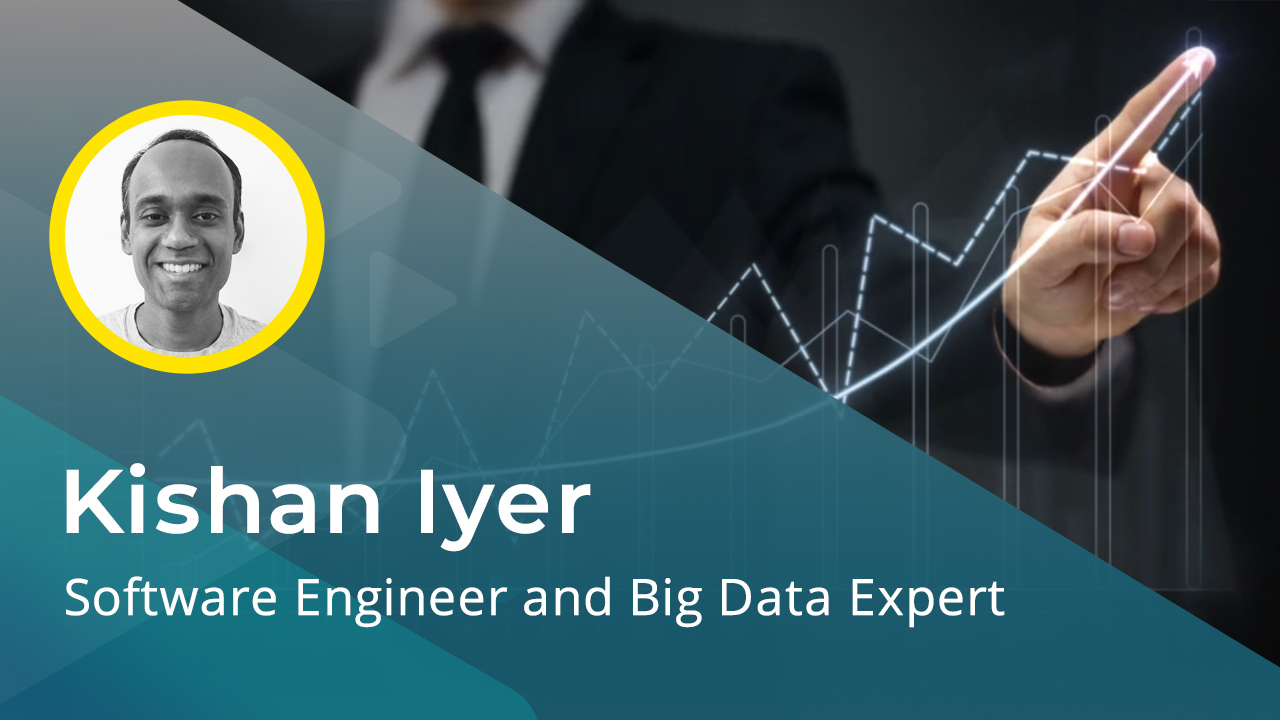Logistic Regression is a technique used to estimate the probability of an outcome. Discover the concepts and explore how logistic regression is used to predict categorical outcomes.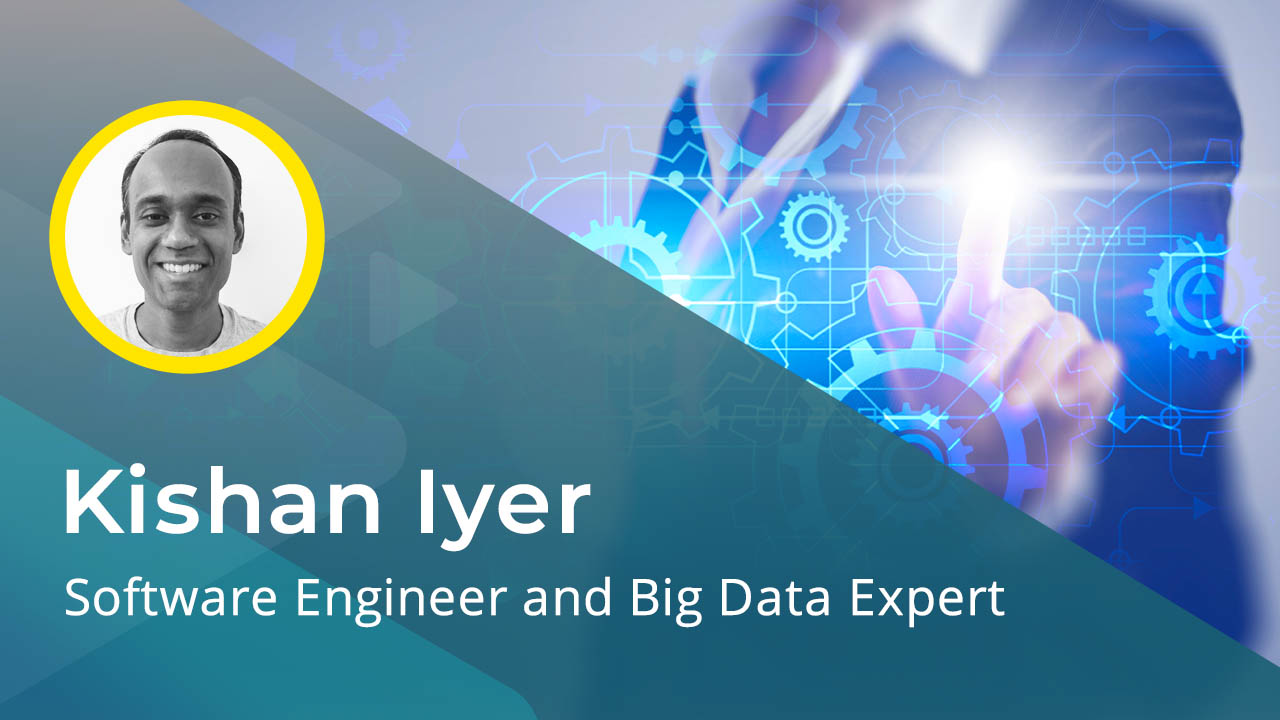Building standard regression and classification models involve a number of steps which are often repetitive and can be standardized. Explore how estimators abstract away a lot of these tasks and speed up the task of model creation.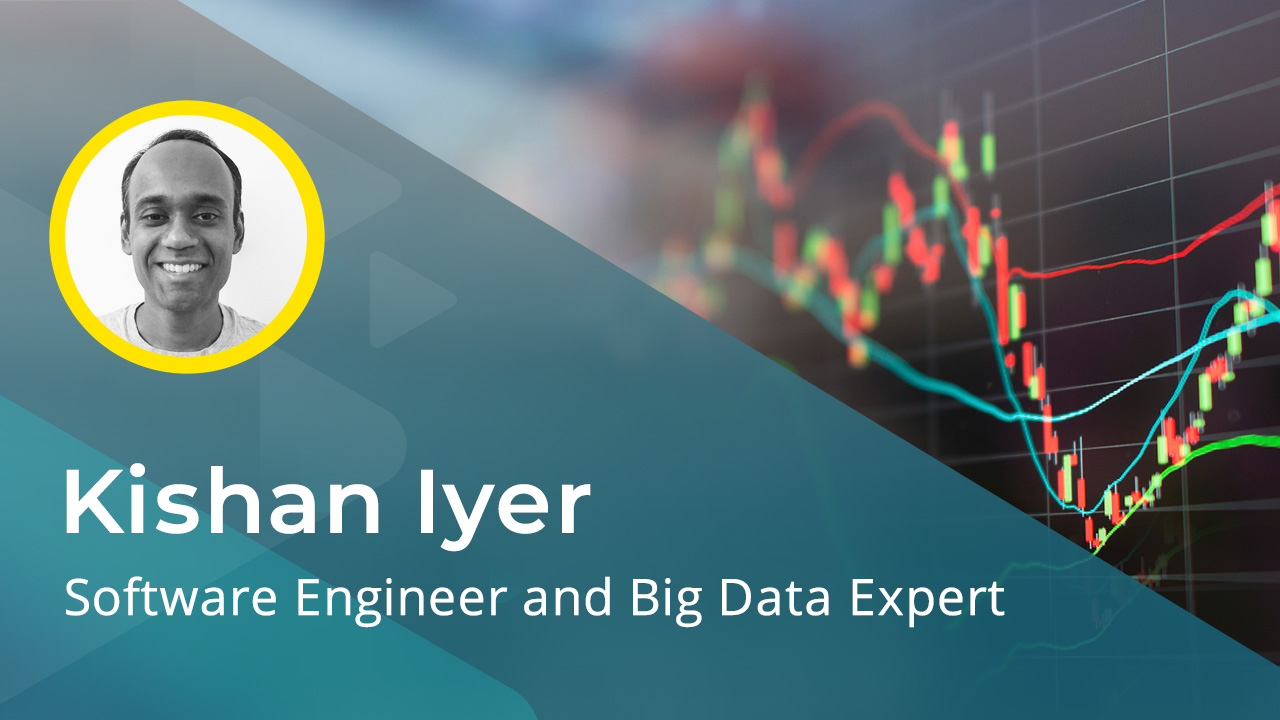There are usually several factors which influence an outcome and we need to consider all of those when trying to predict that event using regression. Explore the techniques and risks involved when using multiple factors for regression.Machine Learning is everywhere these days, often invisible to most of us. Discover one of the fundamental problems in the world of ML - linear regression. Explore how this is solved using classic ML as well as Neural Networks.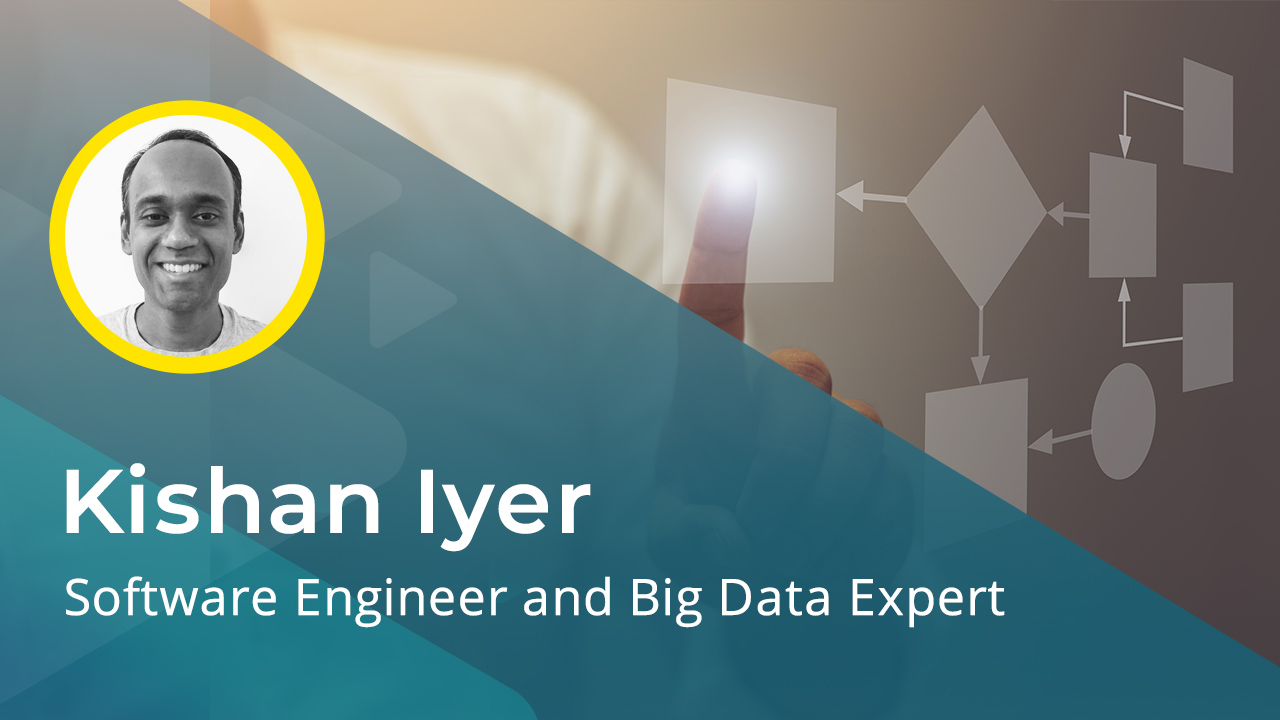Use the Scikit Learn and Keras libraries to build a Linear regression model to predict the price of a house. Understand the steps involved in preparing data and configuring regression models.